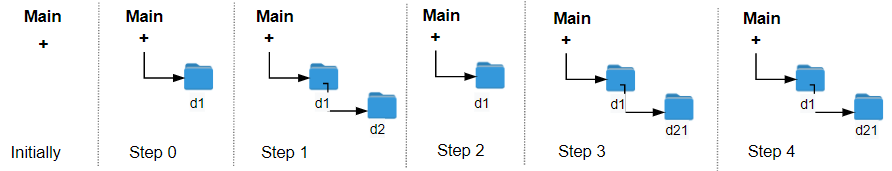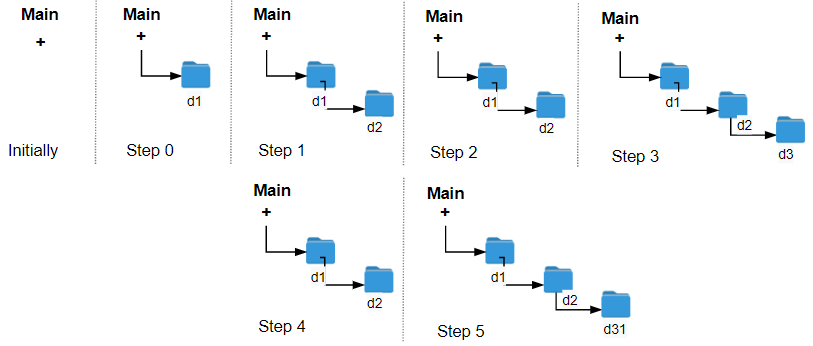Formatted question description: https://leetcode.ca/all/1598.html

# 1598. Crawler Log Folder (Easy)

The Leetcode file system keeps a log each time some user performs a change folder operation.

The operations are described below:

• "../" : Move to the parent folder of the current folder. (If you are already in the main folder, remain in the same folder).
• "./" : Remain in the same folder.
• "x/" : Move to the child folder named x (This folder is guaranteed to always exist).

You are given a list of strings logs where logs[i] is the operation performed by the user at the ith step.

The file system starts in the main folder, then the operations in logs are performed.

Return the minimum number of operations needed to go back to the main folder after the change folder operations.

Example 1:Input: logs = ["d1/","d2/","../","d21/","./"]
Output: 2
Explanation: Use this change folder operation "../" 2 times and go back to the main folder.


Example 2:Input: logs = ["d1/","d2/","./","d3/","../","d31/"]
Output: 3


Example 3:

Input: logs = ["d1/","../","../","../"]
Output: 0


Constraints:

• 1 <= logs.length <= 103
• 2 <= logs[i].length <= 10
• logs[i] contains lowercase English letters, digits, '.', and '/'.
• logs[i] follows the format described in the statement.
• Folder names consist of lowercase English letters and digits.

Related Topics:
Stack

Similar Questions:

## Solution 1.

// OJ: https://leetcode.com/problems/crawler-log-folder/

// Time: O(N)
// Space: O(1)
class Solution {
public:
int minOperations(vector<string>& logs) {
int lv = 0;
for (auto & s : logs) {
if (s == "./") continue;
if (s == "../") lv = max(lv - 1, 0);
else ++lv;
}
return lv;
}
};


Java

class Solution {
public int minOperations(String[] logs) {
int level = 0;
int length = logs.length;
for (int i = 0; i < length; i++) {
String log = logs[i];
if (log.equals("../"))
level = Math.max(level - 1, 0);
else if (log.equals("./"))
continue;
else
level++;
}
return level;
}
}